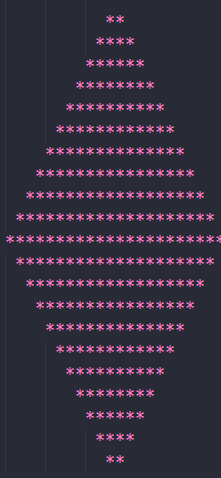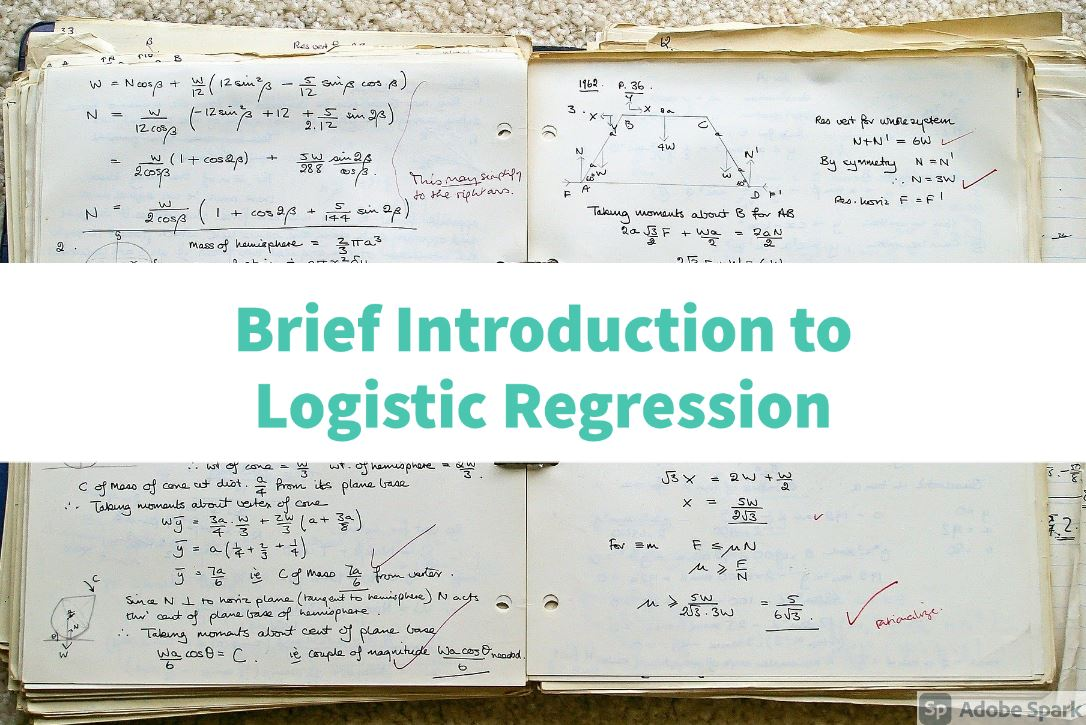# Python 3 Loops and Functions in details

In  last tutorial we have seen how we can iterate in in sequences using foor loop , In this tutorial lets see how we can do some logical stuff using using for loop.

## Basic syntax of for loop

```for i in range(0,10,+2):
pass
```

where 0 is starting index and 10 will be last index and +2 is incremental value.

### Lets try for loop for printing below structure

Now lets create a simple python program to print dimond like structure using for loop,Below picture will be output of our script.So here is our script

```for e in range(0,11,+1):
print((11-e) * ' ' + (2*e)* '*')

for e in range(11,0,-1):
print((11-e) * ' ' + (2*e) * '*')
```

range of first loop is 0,10 (1 less time 11 that is 10) ,

first we have to reduce spaces, for that I used 11-e that means,in first iteration its going to place 11 spaces then 10 then 9 so spaces are going to reduce. after that in same loop and same line multiply * with 2 so that in first row 2 * in second row it print 4 * and vice versa.

In second loop,range is from 10 to 0 because it will be easy if we want to print opposite shape triangle. In first iteration its going to print no space then 1 space then 2 and so on, and in the same line sterics are going to be decreased by 2.

## Functions

Now lets discuss functions so functions are of two types, either they will return some results or they just do processing inside.

Lets define a simple function that take two arguments and return addition of those two variables.

```def main(a,b):
return a+b

result = main(2,3)
print("Result of 2 passed arguments is : ",result)
```

In the same way lets create simple python function that will return square of argument passed in function

```def main(a):
return a**2

print(main(3))
```

You can also pass as many arguments in function as you want, for that use *args as an argument and list of arguments will be created in args variable and you can iterate through it.

```def main(*args):
for i in args:
print(i)

print(main(3,4,"Usman","Alex","Maria"))
```

## Scope of variable

And lastly lets discuss Local and global variable

Global variable is variable on program level and can be accessed at any level in program either in loop or thread or in any class or function.

Local variables are created inside functions or classes or loops and they can only be accessed with in that function or class etc, however local variable of loop can be accessed outside of loop and it will contains the last value at which loop stoped.

### Complete Series